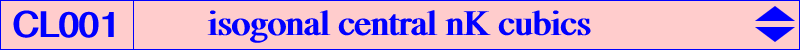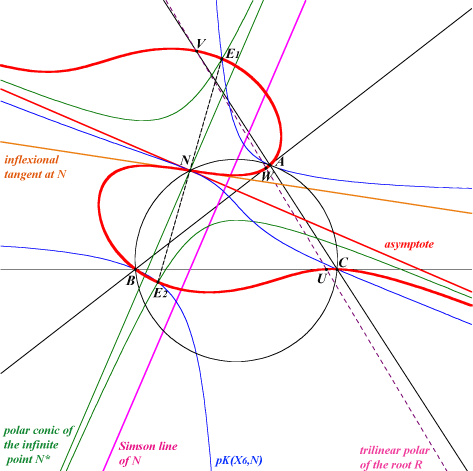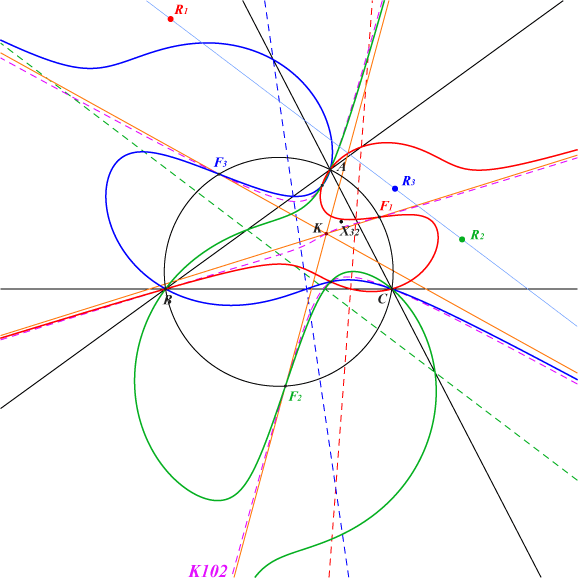All isogonal central nK are circular and have their center N (which is at the same time the singular focus) on the circumcircle. Thus, they are van Rees focal cubics. The general case is detailed in Special Isocubics §3.3. See a generalization in the page P-conical cubics. The real asymptote is the perpendicular at N to the Simson line of N i.e. the line L passing through N and its isogonal conjugate N*. The root R of the cubic is the complement of the isotomic conjugate of Z, trilinear pole of L. R lies on the homothetic of the Simson cubic K010 under h(G,1/4).The polar conic H of the real infinite point N* is a rectangular hyperbola with center N. One of its asymptotes is that of the cubic and the other the perpendicular at N. The curve has two centers of anallagmaty E1 and E2, lying on one bissector of the angle formed by the asymptote and the inflexional tangent. These points lie on the rectangular hyperbola, on pK(X6,N) and are isogonal conjugates. This type of cubic can be seen as the locus of foci of inscribed conics whose center lies on the line L. This line is in fact the Newton line of the quadrangle formed by the sidelines of ABC and the trilinear polar of the root R, meeting the sidelines and the cubic at U, V, W.The points A and U, B and V, C and W share the same tangentials respectively. Hence they are conjugated on the cubic : one of the bisectors of angle (NA, NU) is the real asymptote, the others similarly. The circles with diameters AU, BV, CW belong to the same pencil whose radical axis is the homothetic of the Simson line of N under h(N,2). The cubic obviously passes through the reflections A', B', C', U', V', W' of the mentioned points in N. A' and U', etc are isogonal conjugates. The lines AU' and A'U are parallel to the real asymptote, the others similarly. H contains the midpoints of AU', A'U, etc therefore its construction is easy to realize. With N = X(99), we obtain the cubic K084.   Isogonal central nK0s   When the root R lies on the orthic axis (and on the cubic above), the cubic is a nK0 and its center N is one of the three common points (apart A, B, C) of the Grebe cubic K102 and the circumcircle. The three real asymptotes concur at the Lemoine point K and the tangents at the centers concur at X(32). Recall that the four remaining common points of these three cubics are the foci of the inconic with center K, perspector H.